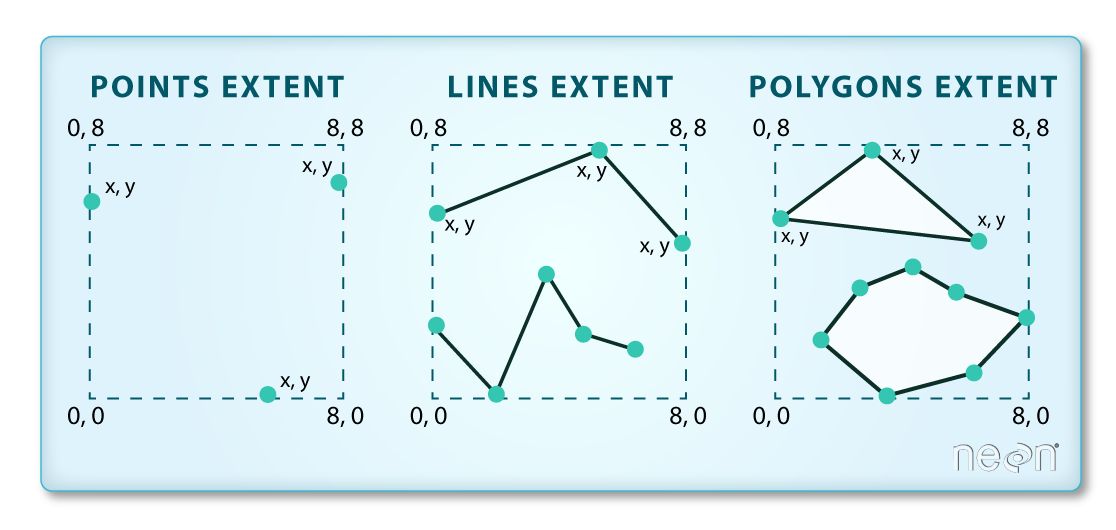# Lesson 3. Customize Map Extents in Python: GIS in Python

## Learning Objectives

• Visually “clip” or zoom in to a particular spatial extent in a plot.

### Import Packages and Data

Begin by importing the necessary packages including geopandas to import the vector data and matplotlib to create the map.

# Import libraries
import os
import matplotlib.pyplot as plt
import geopandas as gpd
import earthpy as et

# Get the data & set working dir
data = et.data.get_data('spatial-vector-lidar')
os.chdir(os.path.join(et.io.HOME, 'earth-analytics', "data"))

# Read in necessary files
"california" ,
"neon-sjer-site",
"vector_data",
"SJER_crop.shp"))

"usa",
"usa-boundary-dissolved.shp"))

"global",


## Change the Spatial Extent of a Plot in Python

Sometimes you modify your data by clipping it to a specified boundary. This is useful when you want to:

1. Make your data smaller to speed up processing and reduce file size.
2. Make analysis simpler and faster given less data to work with.

However, if you just want to plot the data, you can consider adjusting the spatial extent of a plot to “zoom in”.

Note that zooming in on a plot does not change your data in any way - it just changes how your plot renders!

To zoom in on a region of your plot, you first need to grab the spatial extent of the object

# Get spatial extent  - to zoom in on the map rather than clipping
aoi_bounds = sjer_aoi.geometry.total_bounds
aoi_bounds

array([ 254570.567     , 4107303.07684455,  258867.40933092,
4112361.92026107])


The total_bounds attribute represents the total spatial extent for the aoi layer. This is the total external boundary of the layer - thus if there are multiple polygons in the layer it will take the furtherst edge in the north, south, east and west directions to create the spatial extent box.The spatial extent of a shapefile or Python spatial object like a geopandas geodataframe represents the geographic edge or location that is the furthest north, south, east and west. This is the overall geographic coverage of the spatial object. Source: National Ecological Observatory Network (NEON)

The object that is returned is a tuple - a non editable object containing 4 values: (xmin, ymin, xmax, ymax). If you want you can assign each value to a new variable as follows

# Create x and y min and max objects to use in the plot boundaries
xmin, ymin, xmax, ymax = aoi_bounds

fig, ax = plt.subplots(figsize=(14,6))

country_boundary_us.plot(alpha = .5, ax = ax)

ax.set_axis_off()
plt.axis('equal')

plt.show()Plot showing the North American roads overlaid on the continental US without x and y limits being set.

You can set the x and ylimits of the plot using the x and y min and max values from your bounds object that you created above to zoom in your map.

# Use the country boundary to set the min and max values for the plot
country_boundary_us.total_bounds

array([-124.725839,   24.498131,  -66.949895,   49.384358])


Notice in the plot below, you can still see roads that fall outside of the US Boundary area but are within the rectangular spatial extent of the boundary layer. Hopefully this helps you better understand the difference between clipping the data to a polygon shape vs simply plotting a small geographic region.

# Plot the data with a modified spatial extent
fig, ax = plt.subplots(figsize = (10,6))

xlim = ([country_boundary_us.total_bounds,  country_boundary_us.total_bounds])
ylim = ([country_boundary_us.total_bounds,  country_boundary_us.total_bounds])

ax.set_xlim(xlim)
ax.set_ylim(ylim)

country_boundary_us.plot(alpha = .5, ax = ax)